Download Presentation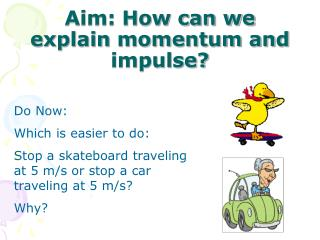Aim: How can we explain momentum and impulse?

# Aim: How can we explain momentum and impulse? - PowerPoint PPT Presentation

Aim: How can we explain momentum and impulse?. Do Now: Which is easier to do: Stop a skateboard traveling at 5 m/s or stop a car traveling at 5 m/s? Why?. Which is easier to do: Stop a bullet fired from a gun or stop a bullet that is thrown at you? Why?. Momentum (p). “Mass in motion”I am the owner, or an agent authorized to act on behalf of the owner, of the copyrighted work described.
Download Presentation## Aim: How can we explain momentum and impulse?

An Image/Link below is provided (as is) to download presentation

Download Policy: Content on the Website is provided to you AS IS for your information and personal use and may not be sold / licensed / shared on other websites without getting consent from its author.While downloading, if for some reason you are not able to download a presentation, the publisher may have deleted the file from their server.

- - - - - - - - - - - - - - - - - - - - - - - - - - E N D - - - - - - - - - - - - - - - - - - - - - - - - - -
Presentation Transcript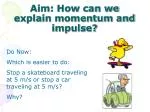### Aim: How can we explain momentum and impulse?

Do Now:

Which is easier to do:

Stop a skateboard traveling at 5 m/s or stop a car traveling at 5 m/s?

Why?Which is easier to do:

Stop a bullet fired from a gun or stop a bullet that is thrown at you?

Why?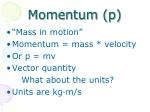Momentum (p)

“Mass in motion”

Momentum = mass * velocity

Or p = mv

Vector quantity

What about the units?

Units are kg·m/s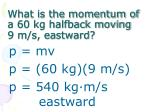p = mv

p = (60 kg)(9 m/s)

p = 540 kg·m/s eastward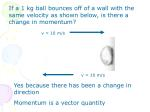If a 1 kg ball bounces off of a wall with the same velocity as shown below, is there a change in momentum?

v = 10 m/s

v = 10 m/s

Yes because there has been a change in direction

Momentum is a vector quantity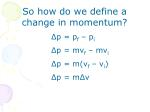So how do we define a change in momentum?

• Δp = pf – pi
• Δp = mvf – mvi
• Δp = m(vf – vi)
• Δp = mΔv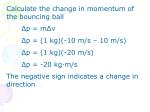Calculate the change in momentum of the bouncing ball

Δp = mΔv

Δp = (1 kg)(-10 m/s – 10 m/s)

Δp = (1 kg)(-20 m/s)

Δp = -20 kg·m/s

The negative sign indicates a change in direction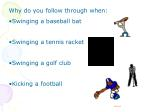Why do you follow through when:

• Swinging a baseball bat
• Swinging a tennis racket
• Swinging a golf club
• Kicking a football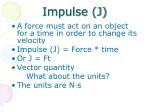Impulse (J)

A force must act on an object for a time in order to change its velocity

Impulse (J) = Force * time

Or J = Ft

Vector quantity

What about the units?

The units are N·s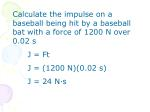Calculate the impulse on a baseball being hit by a baseball bat with a force of 1200 N over 0.02 s

J = Ft

J = (1200 N)(0.02 s)

J = 24 N·sEgg Demo

Why doesn’t the egg break when it hits the bed sheet?We know:

Doesn’t J = Ft?

Doesn’t Δp = mΔv?

So J = Δp

Impulse is a change in momentum!!mΔv is a constant

• mass has not changed
• initial and final velocities have not changed
• Time to slow down increased
• Therefore force has to decrease
• Hence, the egg does not break!Real-World Applications

Baseball and tennis player’s ‘following through’

Boxer’s ‘riding the punch’

AirbagsWhat if force and time are constant but the mass changes?

Astroblaster Demo

If mass decreases, velocity must increase!A car with m=725 kg is moving at 32 m/s to the east. The driver applies the brakes for 2 s. An average force of 5.0 x 103 N is exerted on the car. What is the change in momentum?

Δp = Ft

Δp = (-5 x 103 N)(2 s)

Δp = -1 x 104 N

What is the impulse on the car?

J = Δp

J = -1 x 104 N

What is the car’s final velocity?

Δp = mΔv

-1 x 104 N = (725 kg)(vf – 32 m/s)

-1 x 104 = 725vf – 23,200

vf = 18.2 m/sAn impulse of 30.0 N·s is applied to a 5.00 kg mass. If the mass had a speed of 100 m/s before the impulse, what is its speed after the impulse?

J = mΔv

30 N·s = (5 kg)(vf – 100 m/s)

30 = 5vf – 500

vf = 106 m/sA car with a mass of 1.0 x 103 kg is moving with a speed of 1.4 x 102 m/s. What is the impulse required to bring the car to rest?

J = mΔv

J = (1 x 103 kg)(0 m/s – 1.4 x 102 m/s)

J = -1.4 x 105 N·s